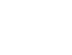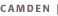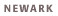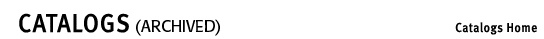CoursesIn the following course list, the Level II Statistics prerequisite may be fulfilled with 01:960: 212 or 384 or 401 or 484 or equivalent. Credit is not given for more than one course fulfilling the Level II Statistics prerequisite. 01:960:211,212Statistics I,II (3,3) Principles and methods of statistics, including frequency distributions, measures of central tendency and dispersion, simple probability, sampling, regression and correlation analysis, curve fitting, chi-square analysis, test of significance. Prerequisite: 01:640:115 or permission of department. See Level II Statistics restrictions. Credit not given for more than one of 01:960:201, 211, and 285, nor for more than one of 01:960:212, 384, and 401. 01:960:285Introductory Statistics for Business (3) Topics include descriptive statistics, probability, random variables, sampling distributions, principles of hypothesis testing, and one and two sample T-tests. Prerequisite: 01:640:115 or equivalent. Credit not given for more than one of 01:960:201, 211, and 285. 01:960:379Basic Probability and Statistics (3) Methods of presenting data; basic statistical measures of location; frequency distributions; elementary probability theory; probability distributions; the binomial, Poisson, and normal distributions; basic sampling theory. Prerequisite: One term of calculus. 01:960:381Theory of Probability (3) Probability distributions; the binomial, geometric, exponential, Poisson, and normal distributions; moment-generating functions; sampling distributions; applications of probability theory. Prerequisites: Three terms of calculus. 01:960:382Theory of Statistics (3) Statistical inference methods, point and interval estimation, maximum likelihood estimators, information inequality, hypothesis testing, Neyman-Pearson lemma, linear models. Pre- or corequisite: 01:960:381 or equivalent. 01:960:384Intermediate Statistical Analysis (3) Application of statistical techniques to the analysis of data, tests of significance, correlation and regression analysis, confidence intervals, analysis of variance, and some design of experiments; analysis of cross-classified data, Chi-square tests. Course requires the use of basic statistics computer package. (Formerly 01:960:380) 01:960:390Introductory Computing for Statistics (1) Introduction to the use of statistics computer packages with main focus on SAS. Includes generating random samples, estimation, testing hypothesis, ANOVA. Five-week course; 3 hrs. lec. and lab. Pre- or corequisite: Level II Statistics. Graded on a Pass/Fail (undergraduate) and S/U (graduate) basis. 01:960:391,392Honors Seminars in Probability/Statistics (3,3) Real-life examples or case studies on statistics and probability theory, and their ramifications. Topics may vary from term to term. Extensive data analysis required. Prerequisite: CALC1 or permission of department. Corequisite: CALC2. Open to students in college honors programs. 01:960:401Basic Statistics for Research (3) As applied in fields other than statistics; treats research projects dependent on the use of observed data from planned experiments. Includes inference methods in estimation and hypothesis testing and general linear models. Prerequisite: 01:640:115 or equivalent. For students in the biological sciences, ceramic engineering, computer sciences, pharmacy, etc. May be followed by 01:960:490, or 16:960:590 with permission of department. Credit not given for more than one of 01:960:212, 384, and 401. 01:960:463Regression Methods (3) Multiple and nonlinear correlation and regression techniques for analysis of events in time and space: analysis of variance and covariance, related multivariate techniques, response surface approaches. Prerequisite: Level II Statistics. 01:960:467Applied Multivariate Analysis (3) Introduction to the methodology of multivariate analysis. Multiple linear regression, discriminant analysis, profile analysis, canonical correlation, principal components, and factor analysis. Prerequisite: Level II Statistics or permission of department. 01:960:476Introduction to Sampling (3) Principles of sampling application for economic procurement or assessment of data. Current techniques for area sampling, sampling of accounts, large-scale surveys, stratification, cluster sampling, systematic sampling, two-stage sampling, and construction of estimates. Prerequisite: Level II Statistics and 01:960:379 or 381 or equivalent or permission of department. 01:960:483Statistical Quality Control (3) Statistical measures; histogram analysis; construction and analysis of control charts for variables and attributes; use of Dodge-Romig and Military Standards acceptance sampling plans; statistical aspects of tolerances. Prerequisite: One term of statistics at the college level. 01:960:484Basic Applied Statistics (3) Confidence estimation, hypothesis testing, chi-square methods, correlation and regression analysis, basis of design of experiments. Prerequisite: One of the following courses: 01:960: 201, 211, 285, 379, 381, or permission of instructor. Credit not given for both this course and 01:960:212 or 384 or 401. 01:960:486Computing and Graphics in Applied Statistics (3) Use of various computer-based techniques, including graphical, to understand and interpret data. Exposure to basic analysis of categorical, time-series, and multivariate data in applied areas such as biostatistics, quality control, and others. 01:960:490Introduction to Experimental Design (3) Basic concept and principles of designs. Nature and analysis of various designs; randomized blocks, Latin squares, factorial designs. Applications to specific problems. Prerequisite: Level II Statistics. 01:960:491Reliability-Quality Control (3) Survey of current theory and practice in this field. Prerequisites: 01:640:251 and Level II Statistics. 01:960:495Independent Studies in Statistics (3) Prerequisite: Permission of department.